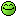# Question: Why does the Gospel of John mention the number 153?

I'd like to know why John records that there were 153 fish caught by the disciples in John 21.

Thanks!

An interested pastor...

Well, before getting into the Bible question, can I tell you a couple interesting facts about the number 153?

First, it's a Triangular Number. That means it's a sum of integers starting with 1, 2, 3, etc. 153 is the 17th Triangular Number, which means it's the sum of all the integers between 1 and 17.

Second, it's a Happy Cube. If you take the digits of 153, cube them, and add them together...

1^3 + 5^3 + 3^3 = 1 + 125 + 27 = 153.

Third, grab any multiple of 3. Let's try 24. Now do the Happy Cube process on 24.

2^3 + 4^3 = 8 + 64 = 72
7^3 + 2^3 = 343 + 8 = 351
3^3 + 5^3 + 1^3 = 153

Pretty cool, huh?

But it doesn't answer your question, which is, why does this number show up in the Gospel of John, chapter 21? The easy answer is: That's how many fish the disciples caught, and accordingly, that's what the Gospel records!

But I know, that doesn't really answer your question, because what you really want to know is, is there any "significance" to that number.

And the Biblical numerologists will have a variety of answers to that. In fact, if you had 153 numerologists, they'd probably have 153 different answers!

Here are a just a couple. And I don't claim that either of these is correct - in fact, I'm usually pretty skeptical about numerological stuff in the Bible (other than clearly stated parallels like the Jonah/Jesus parallel involving the number 3).

One possibility is this: according to one source, if you discount the "mass" miracles (feeding of the 5000, feeding of the 4000) the Gospels record 153 different people that Jesus ministered to 153. So, the 153 is somehow representative of the work Jesus did. I'm pretty skeptical of this, since you could make that count be whatever you wanted it to be, just by counting miracles differently. For example, when Jesus raised Jairus' daughter, was He ministering to Jairus? To his daughter? To both? You see? We can make it add up however we want with just a wave of the hand.

Another possibility, which a friend of mine told me years ago, is this: The number 9 represents the Holy Spirit, and the number 17 represents the uttermost parts of the earth. (Why do these numbers represent these things? That's a good question, and I have no convincing answer!). But if you accept the premise that 9 represents the Holy Spirit, and 17 represents the uttermost parts of the earth, then 153 is 9 x 17, which suggests going to the uttermost parts of the earth by the power of the Holy Spirit.

And there you have just a couple possiblilities. But in my mind, that's all they are, and I haven't seen convincing arguments that either is correct. So when I'm preaching from John 21, I don't make a big deal out of the number.

Wasn't that unhelpful?